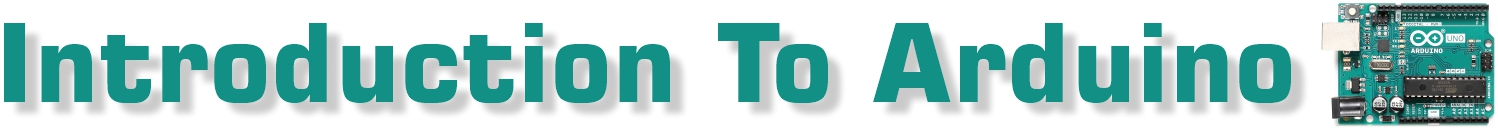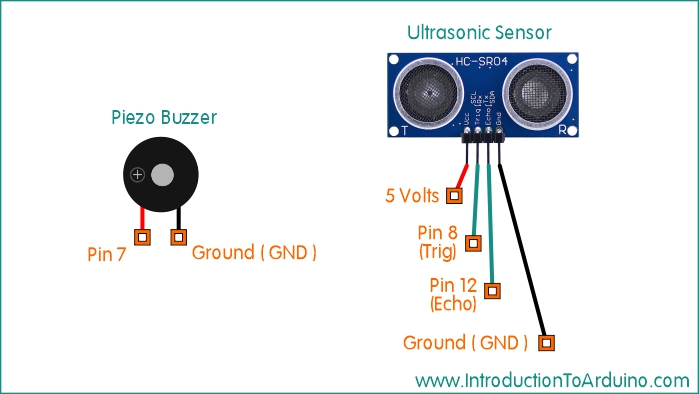Ultrasonic Distance Sensor with Piezo BuzzerArduino UNO R3 Pin Setup Overview: This project uses an HC-SR04 Ultrasonic Sensor to determines the distance to an object. This distance value is passed through a Low Pass Filter to create a smoother stream of values. The filtered data is then used to change the tone of the buzzer according to the object’s distance. Parts List: 1 HC-SR04 Ultrasonic Sensor 1 Small Piezo Buzzer 1 Arduino UNO R3 1 Breadboard Connector Wires Code: ```//------------- Code Starts Here ---------------------------- //----------------------------------------- //Published by IntroductionToArduino.com //Created by Paul Illsley (www.paulillsley.com) //Please use and share so others can enjoy //----------------------------------------- // Declaring pin 8 as the ultrasonic sensor's trigger pin (integer) int trigger_pin = 8; // Declaring pin 12 as the ultrasonic sensor's echo pin (integer) int echo_pin = 12; // Declaring pin 7 as the buzzer pin (integer) int buzzer_pin = 7; // Creating a variable to hold the ultrasonic sensor's data stream (floating point)   float RawData; // Creating a variable to hold information for the data smoothing process // A 0 value is required to start the process (floating point) float OldRawData = 0; // Creating a variable to hold the filtered (smoothed) data (floating point) float FilteredData; // Creating a variable to hold the Distance value (floating point) float Distance; void setup() {  // Begin serial port at a baud rate of 9600  Serial.begin (9600);    // Setting the trigger pin as an output  pinMode(trigger_pin, OUTPUT);    // Setting the echo pin as an input  pinMode(echo_pin, INPUT);    // Setting the buzzer pin as an output  pinMode(buzzer_pin, OUTPUT); } void loop() {  // Setting the trigger pin to low  digitalWrite(trigger_pin, LOW);    // delay 1 microseconds  delayMicroseconds(1);    // Setting the trigger pin to high  digitalWrite(trigger_pin, HIGH);    // delay 5 microseconds  delayMicroseconds(5);    // Setting the trigger pin to low  digitalWrite(trigger_pin, LOW);  // Populating the RawData variable with the echo data from the ultrasonic sensor  // Values are milliseconds between the time the sound pulse left the transmitter and returned back to the receiver  RawData = pulseIn(echo_pin, HIGH);  // Calculating the Distance (cm) from the semsor  Distance = RawData * 0.034 / 2;  // Filter the RawData to help create a smoother data flow.  // The OldRawData is given an 80% weight which is added to the RawData having a 20% weight  // The OldRawData value is derived from original 0 value or created later in the code from the previous loop's FilterdData value  FilteredData=.8*OldRawData+.2*RawData;  // Setting up the serial port's readout format  // Open the Serial Monitor to view your data values (Tools / Serial Monitor)  // Open the Serial Plotter to view your data as a graph (Tools / Serial Plotter)  Serial.print("Raw Data (ms): ");  Serial.print(RawData);  Serial.print(", ");  Serial.print("Distance (cm): ");  Serial.print(Distance);  Serial.print(", ");  Serial.print("Filtered Data (ms): ");  Serial.print(FilteredData);  Serial.println();  // Mapping the buzzer's frequency range for the FilteredData range  // Creating a new variable called Sound (integer)  // Data range is between 50 and 2000  // Frequency range is between 120 and 1500 megahertz  // These values can be changed to suit your data and desired frequency ranges  int Sound = map(FilteredData, 50, 2000, 120, 1500);  // Sending the remapped data (Sound) to pin 7 (the buzzer)  tone(7, Sound, 0);  // Making the variable OldRawData equal to FilteredData to be used in the next loop (for the smoothing process)  OldRawData=FilteredData;  //Delay 10 millisecond (then repeat the loop)  delay(10);         } //------------- Code Stops Here ---------------------------- ``` Created by Paul Illsley Return to www.introductiontoarduino.com